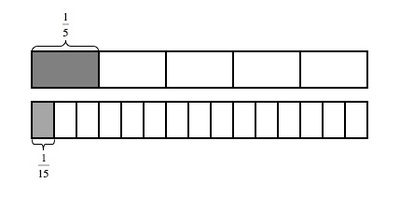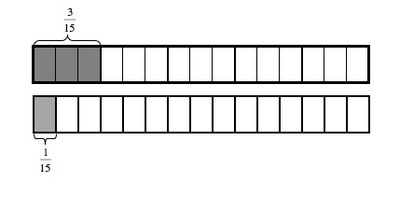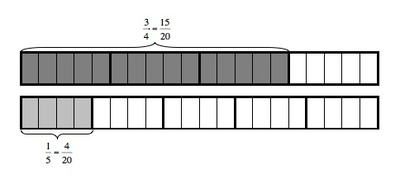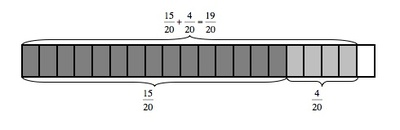# Finding Common Denominators to Add

Alignments to Content Standards: 5.NF.A.1

1. To add fractions, we usually first find a common denominator.
1. Find two different common denominators for $\frac{1}{5}$ and $\frac{1}{15}$.
2. Use each common denominator to find the value of $\frac{1}{5}+\frac{1}{15}$. Draw a picture that shows your solution.

2. Find $\frac{3}{4}+\frac{1}{5}$. Draw a picture that shows your solution.
3. Find $\frac{14}{8}+\frac{15}{12}$.

## IM Commentary

Part (a) of this task asks students to find and use two different common denominators to add the given fractions. The purpose of this question is to help students realize that they can use any common denominator to find a solution, not just the least common denominator. Part (b) does not ask students to solve the given addition problem in more than one way. Instead, the purpose of this question is to give students an opportunity to choose a denominator and possibly to compare their solution method with another student who chose a different denominator. The two most likely student choices are the least common denominator and the product of the two denominators.

Parts (a) and (b) ask students to draw pictures to help them to see why finding a common denominator is an important part of solving the given addition problems. Part (c) does not ask students to draw a picture. Instead, the purpose of part (c) is to give students who are ready to work symbolically a chance to work more efficiently.

The numbers were chosen in each part for a specific reason. Part (a) asks students to add unit fractions, helping to emphasize the need for a common denominator. Additionally, one denominator is a multiple of the other, so students should recognize it as a common denominator for both fractions. Part (b) asks students to add a unit fraction and a non-unit fraction. Part (c) asks students to add fractions where one of the addends is greater than 1. It also gives students a chance to write one of the fractions with a smaller denominator before adding, making the arithmetic that follows easier.

## Solutions

1. The denominators of the fractions in the first problem are 5 and 15. 5 divides evenly into 15, meaning that 15 is a multiple of 5, so 15 itself is a common denominator for the fractions $\frac{1}{5}$ and $\frac{1}{15}$. The picture below shows this:Here is a picture showing the fractions when they are both written in terms of fifteenths.Any multiple of 15 is also a common multiple of 5 and 15. This means that $2\times15=30$ is a common multiple and is a common denominator for the fractions $\frac{1}{5}$ and $\frac{1}{15}$. Thus, 15 and 30 are two different common denominators for the fractions $\frac{1}{5}$ and $\frac{1}{15}$.

2. The first common denominator that we identified in part (i) was 15. Here is a picture that represents $\frac{1}{5}+\frac{1}{15}$:This is how we can write the process of finding this common denominator and adding using symbols:

\begin{align} \frac{1}{5}+\frac{1}{15}&=\frac{1\times3}{5\times3}+\frac{1}{15}\\ &=\frac{3}{15}+\frac{1}{15}\\ &=\frac{3+1}{15}\\ &=\frac{4}{15} \end{align}

The picture shows that after we convert $\frac{1}{5}$ to $\frac{3}{15}$ we can add $\frac{1}{15}$ and we are left with $\frac{4}{15}$, as we found symbolically.

The second common denominator that we identified in part (i) was 30. Here is a picture that represents $\frac{1}{5}+\frac{1}{15}$:This is how we can write the process of finding this common denominator and adding using symbols:

\begin{align} \frac{1}{5}+\frac{1}{15}&=\frac{1\times6}{5\times6}+\frac{1\times2}{15\times2}\\ &=\frac{6}{30}+\frac{2}{30}\\ &=\frac{6+2}{30}\\ &=\frac{8}{30}\\ &=\frac{4\times2}{15\times2}\\ &=\frac{4}{15}\\ \end{align}

The picture shows that after we convert $\frac{1}{5}$ to $\frac{6}{30}$ and $\frac{1}{15}$ to $\frac{2}{30}$ we can add the thirtieths and are left with $\frac{8}{30}$. This solution is equivalent to $\frac{4\times 2}{15\times 2}\frac{4}{15}$, as we found above. Thus, we get the same answer using the two different common denominators that we identified in part (i), as we would expect.

3. In order to find the solution to the addition problem $\frac{3}{4}+\frac{1}{5}$, we can first find a common denominator. 20 is a multiple of 4 and 5 because $4\times5=20$, meaning that 20 is a common denominator for the fractions $\frac{3}{4}$ and $\frac{1}{5}$. Here is a picture that shows these fractions when they are both written in terms of twentieths:Here is a picture that represents $\frac{3}{4}+\frac{1}{5}$:This is how we can write the process of finding a common denominator and adding using symbols:

\begin{align} \frac{3}{4}+\frac{1}{5} &=\frac{3\times5}{4\times5}+\frac{1\times4}{5\times4}\\ &=\frac{15}{20}+\frac{4}{20}\\ &=\frac{15+4}{20}\\ &=\frac{19}{20} \end{align}

The picture shows that when we convert $\frac{3}{4}$ to $\frac{15}{20}$ and $\frac{1}{5}$ to $\frac{4}{20}$ and add twentieths, we have $\frac{19}{20}$, as we found symbolically.

4. In order to solve this addition problem we again find a common denominator for the fractions $\frac{14}{8}$ and $\frac{15}{12}$. 24 is a common multiple for the denominators 8 and 12 because $8\times3=24$ and $12\times2=24$. This means that 24 is a common denominator for the fractions $\frac{14}{8}$ and $\frac{15}{12}$. This is how we can write the process of finding a common denominator and adding using symbols:
\begin{align} \frac{14}{8}+\frac{5}{12} &=\frac{14\times3}{8\times3}+\frac{5\times2}{12\times2}\\ &=\frac{42}{24}+\frac{10}{24}\\ &=\frac{52}{24} \end{align} If we pause for a moment, we will see that $\frac{52}{24}$ is equivalent to $\frac{13}{6}$, although either representation of the sum is correct.

Solution: Alternative approach to part (c)

A student with a robust understanding of fraction equivalence might note that $\frac{14}{8}$ is equivalent to $\frac{7\times 2}{4\times 2}=\frac74$. So:

$$\frac{14}{8}+\frac{5}{12}=\frac74+\frac{5}{12}$$

12 is a common denominator for these two fractions, so:

\begin{align} \frac{14}{8}+\frac{5}{12} &=\frac74+\frac{5}{12}\\ &=\frac{7\times3}{4\times3}+\frac{5}{12}\\ &=\frac{21}{12}+\frac{5}{12}\\ &=\frac{26}{12} \end{align}

Note that $\frac{26}{12}$ is equivalent to $\frac{52}{24}$ as well as $\frac{13}{6}$; any of these answers are acceptable.